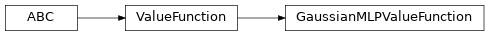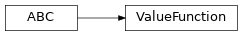# garage.torch.value_functions¶

Value functions which use PyTorch.

class GaussianMLPValueFunction(env_spec, hidden_sizes=(32, 32), hidden_nonlinearity=torch.tanh, hidden_w_init=nn.init.xavier_uniform_, hidden_b_init=nn.init.zeros_, output_nonlinearity=None, output_w_init=nn.init.xavier_uniform_, output_b_init=nn.init.zeros_, learn_std=True, init_std=1.0, layer_normalization=False, name='GaussianMLPValueFunction')Gaussian MLP Value Function with Model.

It fits the input data to a gaussian distribution estimated by a MLP.

Parameters
• env_spec (EnvSpec) – Environment specification.

• hidden_sizes (list[int]) – Output dimension of dense layer(s) for the MLP for mean. For example, (32, 32) means the MLP consists of two hidden layers, each with 32 hidden units.

• hidden_nonlinearity (callable) – Activation function for intermediate dense layer(s). It should return a torch.Tensor. Set it to None to maintain a linear activation.

• hidden_w_init (callable) – Initializer function for the weight of intermediate dense layer(s). The function should return a torch.Tensor.

• hidden_b_init (callable) – Initializer function for the bias of intermediate dense layer(s). The function should return a torch.Tensor.

• output_nonlinearity (callable) – Activation function for output dense layer. It should return a torch.Tensor. Set it to None to maintain a linear activation.

• output_w_init (callable) – Initializer function for the weight of output dense layer(s). The function should return a torch.Tensor.

• output_b_init (callable) – Initializer function for the bias of output dense layer(s). The function should return a torch.Tensor.

• learn_std (bool) – Is std trainable.

• init_std (float) – Initial value for std. (plain value - not log or exponentiated).

• layer_normalization (bool) – Bool for using layer normalization or not.

• name (str) – The name of the value function.

compute_loss(obs, returns)

Compute mean value of loss.

Parameters
• obs (torch.Tensor) – Observation from the environment with shape $$(N \dot [T], O*)$$.

• returns (torch.Tensor) – Acquired returns with shape $$(N, )$$.

Returns

Calculated negative mean scalar value of

objective (float).

Return type

torch.Tensor

forward(obs)

Predict value based on paths.

Parameters

obs (torch.Tensor) – Observation from the environment with shape $$(P, O*)$$.

Returns

Calculated baselines given observations with

shape $$(P, O*)$$.

Return type

torch.Tensor

class ValueFunction(env_spec, name)

Bases: abc.ABC, torch.nn.ModuleBase class for all baselines.

Parameters
• env_spec (EnvSpec) – Environment specification.

• name (str) – Value function name, also the variable scope.

abstract compute_loss(obs, returns)

Compute mean value of loss.

Parameters
• obs (torch.Tensor) – Observation from the environment with shape $$(N \dot [T], O*)$$.

• returns (torch.Tensor) – Acquired returns with shape $$(N, )$$.

Returns

Calculated negative mean scalar value of

objective (float).

Return type

torch.Tensor